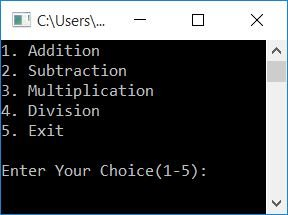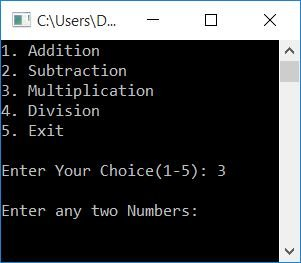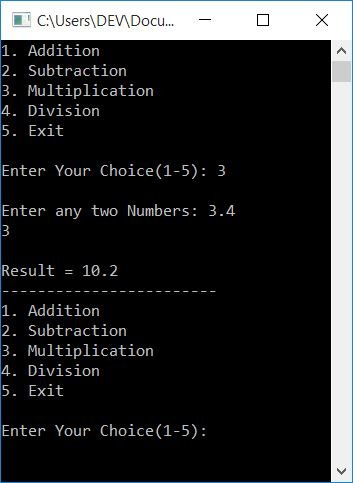# C++ Program to Make a Simple Calculator

In this article, you will learn and get code to create a simple calculator using C++. Here is the list of calculator programs available in this article:

## Make a Calculator in C++

The program given below creates a simple calculator in C++ programming that performs four basic mathematical operations, such as addition, subtraction, multiplication, and division, depending on the user's choice.

To do the task of the calculator, first receive the choice and then any two numbers (if the choice is not to exit). Then, as shown in the program below, use the switch case to identify the choice and perform the necessary actions:

The question is, "How do I write a calculator program in C++?" Here is its answer:

```#include<iostream>
using namespace std;
int main()
{
float numOne, numTwo, res;
int choice;
do
{
cout<<"2. Subtraction\n";
cout<<"3. Multiplication\n";
cout<<"4. Division\n";
cout<<"5. Exit\n\n";
cin>>choice;
if(choice>=1 && choice<=4)
{
cout<<"\nEnter any two Numbers: ";
cin>>numOne>>numTwo;
}
switch(choice)
{
case 1:
res = numOne+numTwo;
cout<<"\nResult = "<<res;
break;
case 2:
res = numOne-numTwo;
cout<<"\nResult = "<<res;
break;
case 3:
res = numOne*numTwo;
cout<<"\nResult = "<<res;
break;
case 4:
res = numOne/numTwo;
cout<<"\nResult = "<<res;
break;
case 5:
return 0;
default:
cout<<"\nWrong Choice!";
break;
}
cout<<"\n------------------------\n";
}while(choice!=5);
cout<<endl;
return 0;
}```

This program was built and runs under the Code::Blocks IDE. Here is its sample run:Now enter your choice, say 3, if you want to perform multiplication. After entering "3" as a selection, press the ENTER key. Here is the output you will see:Now supply any two numbers, say 3.4 and 3, as input. Then press the ENTER key to print its multiplication result, as shown in the snapshot given below:As you can see, the program again asks for the choice to perform the operation again, until the user enters 5 as the choice to exit the calculator.

## Calculator program in C++ using a function

This program also creates a calculator, but using user-defined functions such as:

• addFun() is a function that adds and returns the sum of two numbers passed as arguments.
• subFun() subtracts two numbers. The second number (argument) gets subtracted from the first.
• mulFun() multiplies two numbers.
• divFun() divides two numbers. The first argument gets divided by the second.

Now let's have a look at the program:

```#include<iostream>
using namespace std;
float subFun(float, float);
float mulFun(float, float);
float divFun(float, float);
int main()
{
float numOne, numTwo, res;
int choice;
do
{
cout<<"2. Subtraction\n";
cout<<"3. Multiplication\n";
cout<<"4. Division\n";
cout<<"5. Exit\n\n";
cin>>choice;
if(choice>=1 && choice<=4)
{
cout<<"\nEnter any two Numbers: ";
cin>>numOne>>numTwo;
}
switch(choice)
{
case 1:
cout<<"\nResult = "<<res;
break;
case 2:
res = subFun(numOne, numTwo);
cout<<"\nResult = "<<res;
break;
case 3:
res = mulFun(numOne, numTwo);
cout<<"\nResult = "<<res;
break;
case 4:
res = divFun(numOne, numTwo);
cout<<"\nResult = "<<res;
break;
case 5:
return 0;
default:
cout<<"\nWrong Choice!";
break;
}
cout<<"\n------------------------\n";
}while(choice!=5);
cout<<endl;
return 0;
}
{
return (a+b);
}
float subFun(float a, float b)
{
return (a-b);
}
float mulFun(float a, float b)
{
return (a*b);
}
float divFun(float a, float b)
{
return (a/b);
}```

This program produces the same output as the previous program.

#### The same program in different languages

C++ Quiz

« Previous Program Next Program »